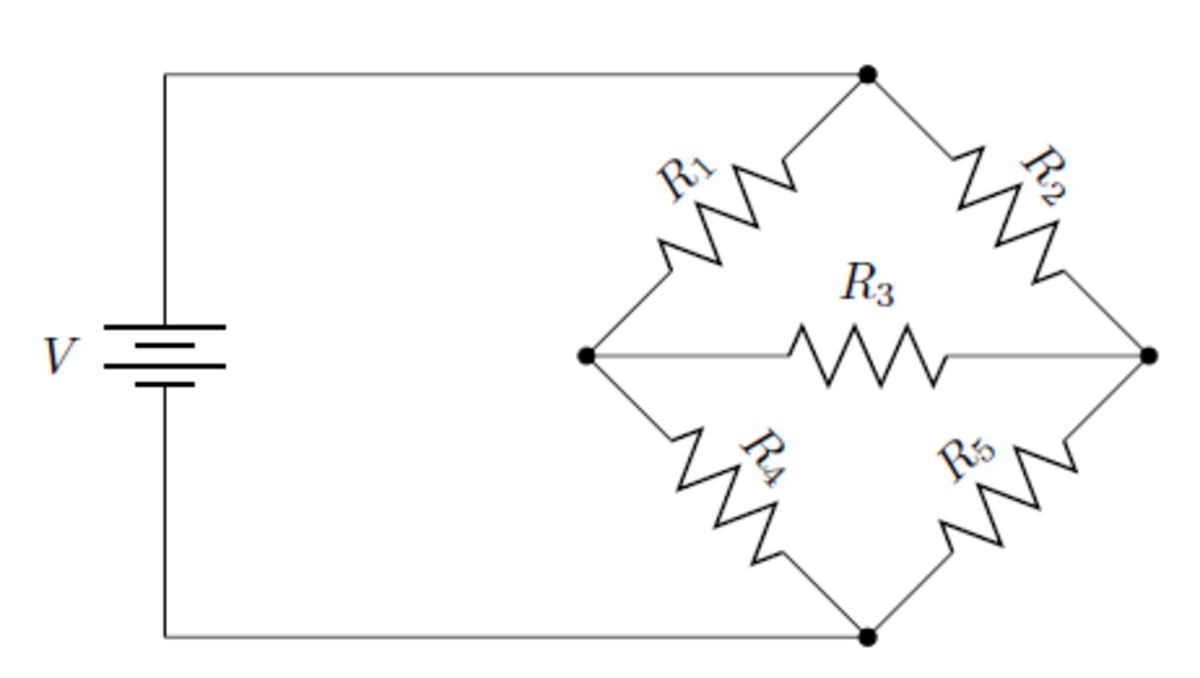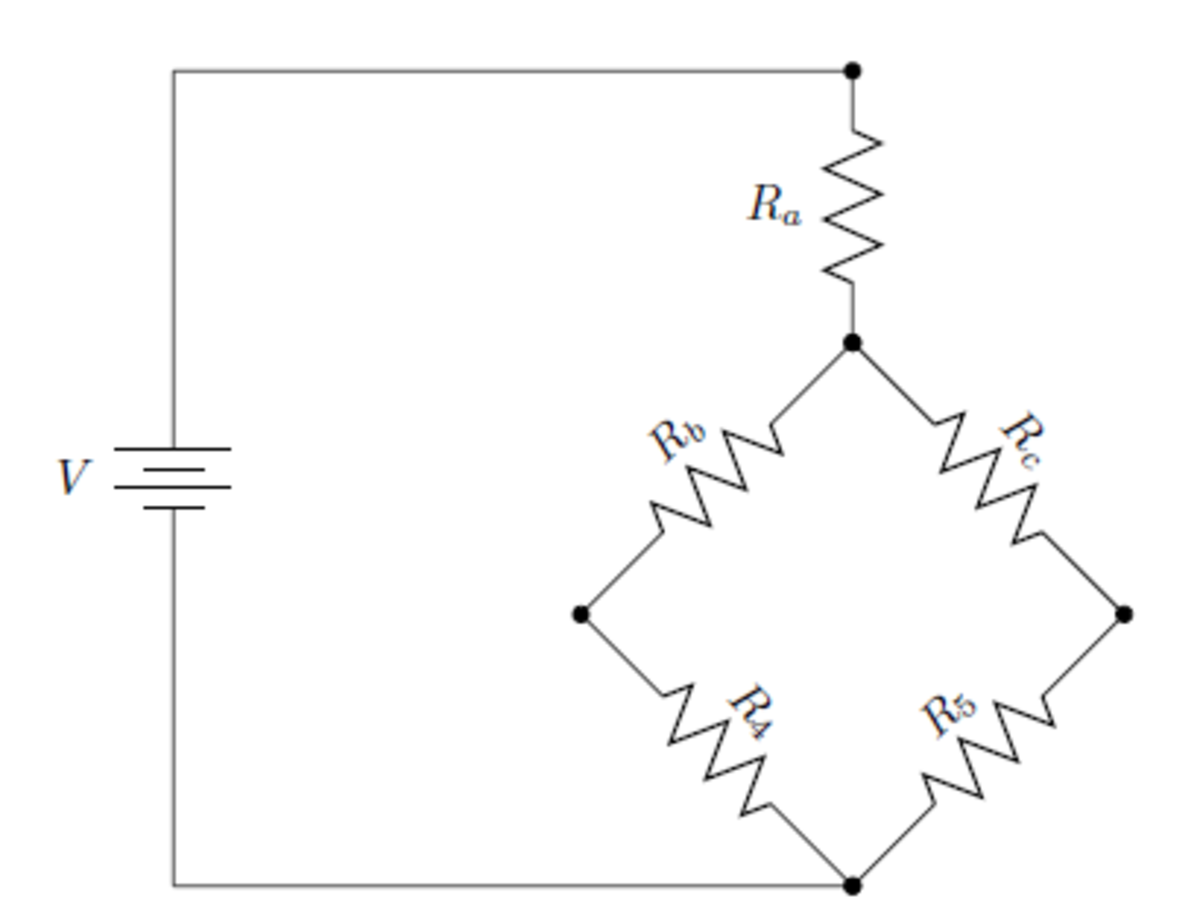# How To Use Delta-to-Wye Transformations In Circuit Analysis

## Theory

In the last Hub, we analyzed a circuit consisting of both series and parallel elements and successfully found the voltage across and current through every resistor using Ohm's Law, Kirchhoff's Voltage and Current Laws, and the formulas to find equivalent resistances for series and parallel circuits. In this Hub, we'll see a circuit orientation that cannot be solved using only these techniques--a delta circuit--and we will see the transformation that makes it possible to solve this type of circuit.

The derivations of the transformations can be found here. To simply state the results, though, if you have a delta circuit and wye circuit as shown above, then the following equations apply:

• Ra = (R1R3) / (R1 + R2 + R3)
• Rb = (R1R2) / (R1 + R2 + R3)
• Rc = (R2R3) / (R1 + R2 + R3)

A pattern is easily observable. The value of a given limb resistor in the wye configuration is equal to the product of the touching corner resistors divided by the sum of the three.

## Application

Let's look at an example circuit shown below:This circuit can't be simplified with only series and parallel substitutions.Pete Michner

What we can do to simplify this resistive network down to a single resistor value is convert the Delta network formed by R1, R2, and R3 into an equivalent Wye network as shown in the following circuit:The equivalent circuit of the previous circuit. Now, one only has to calculate what Ra, Rb, and Rc are.Pete Michner

This circuit is easily solvable using the principles covered in previous hubs. Ra and R4 are in series, and this subbranch is in parallel with the series subbranch of Rc and R5. And these two branches in parallel are in series with Ra.

Using the notation '||' to represent the product-over-sum operation, we have Req = Ra + ( (Rb + R4) || (Rc + R5) ).

Now we use the Delta-Wye transformation. We can't use the exact formulas as written above, since I labeled Ra, Rb, and Rc slightly differently relative to R1, R2, and R3 compared to how I labeled them in the paragraphs at the top of this Hub. But remember the way to arrive at the proper formula: for a given limb on the Wye circuit, divide the two "touching" resistors of the Delta formation by the sum of the three Delta resistors.

So we have:

• Ra = (R1R2) / (R1 + R2 + R3)
• Rb = (R1R3) / (R1 + R2 + R3)
• Rc = (R2R3) / (R1 + R2 + R3)

That's it! Substituting these calculated values in for Ra, Rb, and Rc in the equation for Req above gets us Req, which we can use to calculate the total current draw IT in the circuit.

## An Example Circuit

Let's use the techniques described above to fine the calculated values of the voltage across and current through every resistor in a real circuit, and then compare those calculated values to actually measured values. Shown below is a real circuit in the same configuration as the circuit above with resistors R1-R5:

In this circuit, the nominal battery and resistor values are V = 1.5 V, R1 = 100 kΩ, R2 = 1.2 kΩ, R3 = 1.5 kΩ, R4 = 210 Ω, and R5 = 210 Ω (I didn't purposely the bottom two resistors the same value; I just picked what was already on my breadboard. Also, it doesn't make the solution any kind of simplified special case.)

I like using a spreadsheet program like Microsoft Excel or OpenOffice Calc to lay out circuit equations because if you ever change a resistor value, all the others that depend on the one you just changed automatically recalculate.

Scroll to Continue

To start, I insert the resistor values R1 - R5 into some cells as shown:

Next, we want the spreadsheet to calculate the values of Ra, Rb, and Rc according to the formulas given above. Given the current state of the spreadsheet, we have

• Ra = B1 * B2 / (B1 + B2 + B3)
• Rb = B1 * B3 / (B1 + B2 + B3)
• Rc = B2 * B3 / (B1 + B2 + B3)

The spreadsheet now looks like this:

And the spreadsheet value for Req comes out to be

• Req = B6 + ( (B7 + B4) * (B8 + B5) ) / (B7 + B4 + B8 + B5)

Now the spreadsheet looks like this:

Next, we add VT = 1.5 V to a cell in the spreadsheet. Now we can work through the regular series-parallel circuit shown in the second schematic above. IT, the total circuit current, is VT / Req. With the current values, this comes out to approximately 1.1 mA. This is the current that flows through Ra, making the voltage drop across Ran equal to RaIT, which is approximately 1.28 V (much of the battery's 1.5 V ).

We can label the remainder voltage that exists across the two branches VRem. This is of course VT - Va, which is approximately 0.22 V.

What we want to figure out are the voltage levels at the point between Rb an,d R4, which we can call Rb4, and at the point between Rc and R5, which we can call Rc5.

We can calculate the current flowing through the branch of Rb and R4 , which we can label Ib4, by dividing VRem by Rb + R4, which comes out to approximately 0.13 mA. With this much current flowing through this branch, and therefore through R4, the voltage of Rb4 with respect to the battery's negative terminal is Ib4R4, which we can call VR4, is approximately 0.028 V.

We can calculate the current flowing through the branch of Rc and R5, which we can label Ic5, by dividing VRem by Rc + R5, which comes out to approximately 0.97 mA. (Notice the sanity check we can do on our equations at this point: because of Kirchhoff's Current Law, Ic5 and Ib4 should add up to IT, which they do). With this much current flowing through this branch, and therefore through R5, the voltage of Rc5 with respect to the battery's negative terminal is Ic5R5, which we can call VR5, is approximately 0.20 V.

Now since the Wye version of the circuit is equivalent to the Delta version, we can say that the voltage potential across R3 is equal to the potential difference between VR4 and VR5. Current flows from a higher voltage to a lower voltage, so in this given circuit the current through R3 flows in the direction from right to left since VR5 > VR4. The voltage across R3, which we can call VR3, is VR5- VR4, which is approximately 0.17 V. And the current through R3 is VR3 / R3, which is approximately 0.12 mA.

The last things to calculate are the voltages across and the currents through R1 and R2.

The voltage at the very top node with respect to the negative battery terminal is of course VT, which is 1.5 V, and we know that the voltage at the node at the top of R4 with respect to the negative battery terminal is approximately 0.028 V, so the difference between these two voltages is the voltage VR1, which is approximately 1.47 V. The current IR1 is simply the voltage across it divided by the resistance, which is approximately 1.47E-05 A (not surprising that this current is so small, given that this resistor is so large).

The voltage across R2 is VT - VR5 which is approximately 1.30 V, and the current through R2 is that voltage divided by its resistance, which comes out to approximately 1.1 mA.

An image of my final spreadsheet is shown to the right.

All the calculated and nominal voltages, resistances, and currents and the measured values are shown in the table below:

ValueNominal/CalculatedMeasured% Error

VT

1.50 V

1.40 V

7.1

R1

100 kΩ

98.9 kΩ

1.1

R2

1.20 kΩ

1.18 kΩ

1.7

R3

1.50 kΩ

1.48 kΩ

1.4

R4

210 Ω

217 Ω

3.2

R5

210 Ω

216 Ω

2.8

VR1

1.47 V

1.37 V

7.3

VR2

1.30 V

1.20 V

8.3

VR3

175 mV

168 mV

4.2

VR4

27.6 mV

27.7 mV

0.36

VR5

203 mV

197 mV

3.0

IT

1.10 mA

967 μA

14

IR1

14.7 μA

13.7 μA

7.3

IR2

1.08 mA

955 μA

13

IR3

117 μA

108 μA

8.3

IR4

131 μA

121 μA

8.3

IR5

965 μA

821 μA

18

Most of the calculated values are pretty close to the measured values.

Hopefully the working out of this sample circuit showed you some useful techniques for circuit analysis. The best way to increase your skills is to get lots of practice!